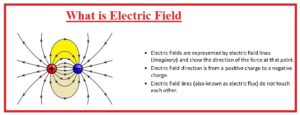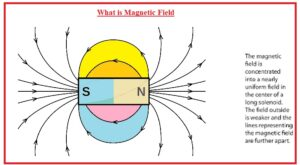Hello, readers welcome to the new post. Here we will discuss Difference between Electric & Magnetic Field. The basic difference is that the magnetic field is produced across the moving charges or about the poles north and south of the magnet and the electric field produces about the static charges.

Here we will discuss different parameters to find the differences among them. So let’s get started Difference between Electric & Magnetic fields.

### Difference between Electric & Magnetic Field

What is Electric Field

• The electric field is a field that exists about the static charges and applies forces on the other charges existing near the field attracting and repelling force• It is also called the physical field for the charged system.
• It produces from the electric charges and through changing magnetic fields.
• Electric and magnetic fields are fundamental forces of nature.
• It is commonly used in different physics modules and is considered part of electrical engineering.
• In the case of atomic, it is an attractive force and used to holds the nucleus and electrons.
• The chemical bond existing between the molecules caused by this force
• SI unit of this force is volt per meter and the same to N/C
• Electric field denoted as E
• The magnetometer is used for its measurement
• The direction of the electric field is at ninety degrees to an electric field
• It produces through positive charges and end at negative charges or point
• For of attraction exist between different charges and attraction exists between same charges
• It is two-dimensional forces
• The equation of electric fields E=F/Q

### What is Magnetic Field• Magnetic files exist about the moving charges or current and magnetic materials
• The movable charges in the field bear the forces at ninety degrees to their velocity and the magnetic field
• The magnetic field of a permanent magnet repels ferromagnetic materials like iron and attracts or repels other types of magnetic materials
• The magnetic field that changes on the basis of location applies force at non-magnetic materials by disturbing the movement of their external electrons.
• It is a vector quantity
• For electromagnetics magnetic field is employed for 2 different but related vectors fields with symbolic representations B and H.
• H is magnetic field strength is calculated in units amps per meter. B is magnetic flux density finds in tesla.
• Tesla is equal to newton per meter per ampers.
• A magnetic file is created through moveable electric charges.
• It is denied as B
• ELectrometer is used for measuring the magnetic field
• It is at ninety degrees to the electrical field
• It is generated through the north pole of the magnet and ends at the south pole
• It works in three dimensions

That is all about the Difference between Electric & Magnetic Field all details has been explained if you have any question ask in the comments.